# Resources tagged with: Place value

Filter by: Content type:
Age range:
Challenge level:

There are 118 NRICH Mathematical resources connected to Place value, you may find related items under Numbers and the Number System.

Broad Topics > Numbers and the Number System > Place value##### Age 5 to 11 Challenge Level:

Try out this number trick. What happens with different starting numbers? What do you notice?##### Age 5 to 11 Challenge Level:

Who said that adding couldn't be fun?### Subtraction Surprise

##### Age 7 to 14 Challenge Level:

Try out some calculations. Are you surprised by the results?### Multiply Multiples 3

##### Age 7 to 11 Challenge Level:

Have a go at balancing this equation. Can you find different ways of doing it?### Round the Dice Decimals 1

##### Age 7 to 11 Challenge Level:

Use two dice to generate two numbers with one decimal place. What happens when you round these numbers to the nearest whole number?### Round the Dice Decimals 2

##### Age 7 to 11 Challenge Level:

What happens when you round these numbers to the nearest whole number?### Multiply Multiples 2

##### Age 7 to 11 Challenge Level:

Can you work out some different ways to balance this equation?### Multiply Multiples 1

##### Age 7 to 11 Challenge Level:

Can you complete this calculation by filling in the missing numbers? In how many different ways can you do it?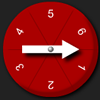### Two Spinners

##### Age 5 to 7 Challenge Level:

What two-digit numbers can you make with these two dice? What can't you make?### Light the Lights

##### Age 5 to 7 Challenge Level:

Investigate which numbers make these lights come on. What is the smallest number you can find that lights up all the lights?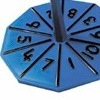### Our Numbers

##### Age 5 to 7 Challenge Level:

These spinners will give you the tens and unit digits of a number. Can you choose sets of numbers to collect so that you spin six numbers belonging to your sets in as few spins as possible?### Coded Hundred Square

##### Age 7 to 11 Challenge Level:

This 100 square jigsaw is written in code. It starts with 1 and ends with 100. Can you build it up?##### Age 11 to 14 Challenge Level:

Powers of numbers behave in surprising ways. Take a look at some of these and try to explain why they are true.### What Do You Need?

##### Age 7 to 11 Challenge Level:

Four of these clues are needed to find the chosen number on this grid and four are true but do nothing to help in finding the number. Can you sort out the clues and find the number?##### Age 7 to 14 Challenge Level:

Watch our videos of multiplication methods that you may not have met before. Can you make sense of them?### Diagonal Sums

##### Age 7 to 11 Challenge Level:

In this 100 square, look at the green square which contains the numbers 2, 3, 12 and 13. What is the sum of the numbers that are diagonally opposite each other? What do you notice?### How Many Miles to Go?

##### Age 11 to 14 Challenge Level:

How many more miles must the car travel before the numbers on the milometer and the trip meter contain the same digits in the same order?### What an Odd Fact(or)

##### Age 11 to 14 Challenge Level:

Can you show that 1^99 + 2^99 + 3^99 + 4^99 + 5^99 is divisible by 5?### Trebling

##### Age 7 to 11 Challenge Level:

Can you replace the letters with numbers? Is there only one solution in each case?### Sixty-seven Squared

##### Age 16 to 18 Challenge Level:

Evaluate these powers of 67. What do you notice? Can you convince someone what the answer would be to (a million sixes followed by a 7) squared?### Six Times Five

##### Age 11 to 14 Challenge Level:

How many six digit numbers are there which DO NOT contain a 5?### Which Is Quicker?

##### Age 7 to 11 Challenge Level:

Which is quicker, counting up to 30 in ones or counting up to 300 in tens? Why?### Reach 100

##### Age 7 to 14 Challenge Level:

Choose four different digits from 1-9 and put one in each box so that the resulting four two-digit numbers add to a total of 100.### All the Digits

##### Age 7 to 11 Challenge Level:

This multiplication uses each of the digits 0 - 9 once and once only. Using the information given, can you replace the stars in the calculation with figures?### Number Rules - OK

##### Age 14 to 16 Challenge Level:

Can you convince me of each of the following: If a square number is multiplied by a square number the product is ALWAYS a square number...### Two and Two

##### Age 11 to 14 Challenge Level:

How many solutions can you find to this sum? Each of the different letters stands for a different number.### Which Scripts?

##### Age 7 to 11 Challenge Level:

There are six numbers written in five different scripts. Can you sort out which is which?### Plus Minus

##### Age 14 to 16 Challenge Level:

Can you explain the surprising results Jo found when she calculated the difference between square numbers?### Legs Eleven

##### Age 11 to 14 Challenge Level:

Take any four digit number. Move the first digit to the end and move the rest along. Now add your two numbers. Did you get a multiple of 11?### Big Powers

##### Age 11 to 16 Challenge Level:

Three people chose this as a favourite problem. It is the sort of problem that needs thinking time - but once the connection is made it gives access to many similar ideas.### 2-digit Square

##### Age 14 to 16 Challenge Level:

A 2-Digit number is squared. When this 2-digit number is reversed and squared, the difference between the squares is also a square. What is the 2-digit number?### Writing Digits

##### Age 5 to 7 Challenge Level:

Lee was writing all the counting numbers from 1 to 20. She stopped for a rest after writing seventeen digits. What was the last number she wrote?### The Number Jumbler

##### Age 7 to 14 Challenge Level:

The Number Jumbler can always work out your chosen symbol. Can you work out how?### Being Curious - Lower Primary Number

##### Age 5 to 7

Number problems to spark your curiosity.### Song Book

##### Age 7 to 11 Challenge Level:

A school song book contains 700 songs. The numbers of the songs are displayed by combining special small single-digit cards. What is the minimum number of small cards that is needed?### Being Resilient - Primary Number

##### Age 5 to 11 Challenge Level:

Number problems at primary level that may require resilience.### Being Resourceful - Primary Number

##### Age 5 to 11 Challenge Level:

Number problems at primary level that require careful consideration.### Being Collaborative - Primary Number

##### Age 5 to 11 Challenge Level:

Number problems at primary level to work on with others.### Being Curious - Primary Number

##### Age 5 to 11 Challenge Level:

Number problems for inquiring primary learners.### More Upper Primary Activities on Number Sense and Place Value

##### Age 7 to 11

More upper primary number sense and place value tasks.### More Lower Primary Number Sense and Place Value Activities

##### Age 5 to 7

More activities which will help you get a better of sense of numbers and understand what we mean by place value.### Amount

##### Age 5 to 11

These tasks will help learners develop their understanding of place value, particularly giving them opportunities to express numbers as amounts.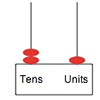### Position

##### Age 5 to 11

One of the key ideas associated with place value is that the position of a digit affects its value. These activities support children in understanding this idea.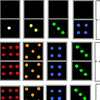### Ordering

##### Age 5 to 11

This set of activities focuses on ordering, an important aspect of place value.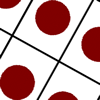### Ten-ness of Ten

##### Age 5 to 7

These tasks will help children understand the 'ten-ness' of ten, a fundamental part of place value.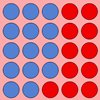### Place Value as a Building Block for Developing Fluency in the Calculation Process

##### Age 5 to 11

This article for primary teachers encourages exploration of two fundamental ideas, exchange and 'unitising', which will help children become more fluent when calculating.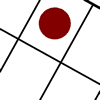### Ten-frames Games

##### Age 3 to 7

These games use ten-frames to develop children's 'sense of ten'.### Activities on the Gattegno Chart

##### Age 5 to 11

In this article, Alf outlines six activities using the Gattegno chart, which help to develop understanding of place value, multiplication and division.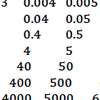### How Can I Support the Development of Early Number Sense and Place Value?

##### Age 3 to 11

This article for primary teachers expands on the key ideas which underpin early number sense and place value, and suggests activities to support learners as they get to grips with these ideas.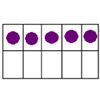### Place Value: the Ten-ness of Ten

##### Age 3 to 11

This article develops the idea of 'ten-ness' as an important element of place value.